I am using a 5% position size for my trading now.

On day 0 I run a backtest, place the quotes in a quote window, and it autoplaces the trades buying x number of shares for a given stock.

On day 1 I run the backtest after the market closes, I sort the signals by type, then name, then Action which puts all the signals in alphabetical order and keeps the sell orders up top and the OCO orders together. (Thanks for making the sorts sticky). Then I highlight all the sell orders and press place.

Ideally, this would be all I need to do. Unfortunately what I'm finding out is that the signals don't match with the previous day's signals. On day 0 it might buy 52 shares of XYZ, but then when I go to place the sell order it wants to sell 54 shares.

So I have to have the accounts window open next to the Signals window, edit every signal that has an incorrect number of shares, and then place the orders.

How is it that the signals differ from day to day? Shouldn't the size of the position be determined by the equity at the start of the trading day? Or is the position size being determined based on a random (or time based) order of executions and the equity being used is after each trade rather than the start of the day equity?

If it's the latter and not a bug, I'll open a feature request to have it be based on the start of the day.

I'm trying to have manual intervention free trading but there are still a few hiccups.

Thanks
0
763
Solved
23 Replies

Bookmark

Sort
There are a few reasons the signal quantity differs day to day.

First off, if you're using a date range like Recent N Years for example, your starting date is continually advancing. That means earlier trades will drop off, resulting in a different ending equity off which to base the calculation.

Another possible reason is NSF positions, but I think by now you're aware of how to deal with them.

To avoid this in my personal live trading, I stick to a Fixed Amount position size and never use Pct of Equity.
1
Hi,

I have it fixed start date and an end date in 2030 so it's always the same.

I also don't have any NSF positions.

So unfortunately those don't explain it.

I'm really trying to get to a point where it's mindless for me to use WL. That includes increasing or decreasing position sizes with account changes over time. It especially includes close order entry with the same position size as open orders that were placed.

Thoughts? I'm sorry I don't consider this solved yet and still consider it to be a possible bug.
0
Something must be causing the size change, maybe you are applying data changes or corrections? I can't think of anything else off the top of my head, but I'll start a daily strategy Monday using percent of equity and report back my findings after a few days.
0
Thank you.

It turns out you don't even necessarily need to wait.

I ran the startegy with an end date of Thursday and looked at the signals. They matched the actual positions in the account. I.e. they are the same signals placed on Thursday and are consistent.

I then ran it in a separate window to end in 2099 (i.e. Friday) and the sell signal position sizes don't match the buy signals from the same strategy.

Thanks for not dismissing this.
0
I think we'll need to see your Strategy, because in my runs the signal quantities are consistent.

You can email it to support@wealth-lab.com with all the relevant settings.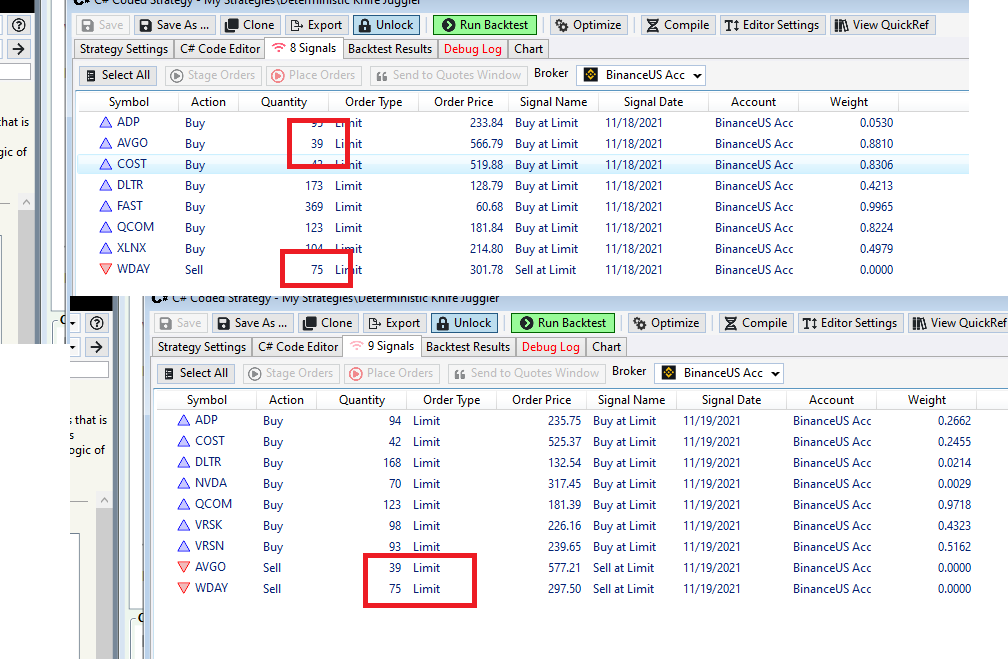0
or if you don’t want to send it, we will need a detailed minimal example that illustrates the issue.

For example, Strategy 50/20 Crossover in Sample Strategies, start date 1/1/2020 position size 5%, starting capital 100000. with end date set to 11/18/2021 buy signal for XYZ is 1234 shares. when end date changed to 11/19/2021 position XYZ has actually 2345 shares.
0
Here's some sample code:

```CODE:using WealthLab.Backtest;
using System;
using WealthLab.Core;
using WealthLab.Indicators;
using System.Drawing;
using System.Collections.Generic;

namespace WealthScript1
{
public class HelloWorldStrategy : UserStrategyBase
{
//declare parameter variables
int max_days;
double profit_target, stop_loss;
int percent_sma;

//declare other variables

//time series variables are used for calculated bar data
TimeSeries sma_values;

//the base procedure must have the same name as the class
public HelloWorldStrategy() : base()
{
AddParameter("Profit Target", ParameterTypes.Double, 2, 1, 3, 1);
AddParameter("Stop Loss", ParameterTypes.Double, 2, 1, 3, 0.5);
AddParameter("Max Days", ParameterTypes.Int32, 2, 2, 5, 1);
AddParameter("Percent SMA", ParameterTypes.Int32, 5, 5, 100, 5);

}

//create indicators and other objects here, this is executed prior to the main trading loop
//also do plotting here
public override void Initialize(BarHistory bars)
{

//assign parameters to variables
profit_target = (double)Parameters.Value;
stop_loss = (double)Parameters.Value;
max_days = (int)Parameters.Value;
percent_sma = (int)Parameters.Value;

//create new series that can be pre-calculated here
sma_values = SMA.Series(bars.Close, 14);

//create new series that need to be calculated here

//ignore data before the StartIndex
StartIndex = 1;

//put headers on the chart here

//do all the plotting of anything that can be pre-calculated here
//   plot anything that needs to be calculated below in Cleanup
PlotTimeSeries(sma_values, sma_values.Description, "Price", Color.Red, PlotStyles.Line);

}

//color the background based on the level of profit/loss
public void WinLossBackground(BarHistory bars, int idx)
{
int profit_level = 0;
System.Drawing.Color color;
// use code_s to find the worst trade for overlapping positions
double profit = 0.0, code_s = 0.0;

// First determine the worst profit level at each bar based on the positions
List<Position> positions = GetPositions();

foreach (Position p in positions)
{
// foreach (Position p in OpenPositions) won't work because it skips the ExitBar
if (!(p.IsOpen || idx == p.ExitBar))
continue;

//can't use p.Profit because the profit isn't calculated yet so it just gives open cost
profit = p.ProfitPctAsOf(idx);

// Profit/Loss in percent
if ((profit > 5))
profit_level = (int)-1;
else if ((profit > 0.5))
profit_level = (int)-2;
else if ((profit > -0.5))
profit_level = (int)-3;
else if ((profit > -5))
profit_level = (int)-4;
else
// profit <= -5.0
profit_level = (int)-5;

double old_code = 0.0;

// keep most pessimistic in overlapping trades
old_code = (double)code_s;
if ((profit_level < old_code))
code_s = profit_level;
} // foreach position

// Now set the background color for the bar
int color_code = 0;

color_code = (int)Math.Floor(code_s);
switch (color_code)
{
case -1:
color = Color.LimeGreen;
break;
case -2:
color = Color.LightGreen;
break;
case -3:
color = Color.LightYellow;
break;
case -4:
color = Color.MistyRose;
break;
case -5:
color = Color.LightPink;
break;
default:
color = Color.White;
break;
} // switch   color_code

SetBackgroundColor(bars, idx, color);
} // WinLossBackground

double RoundPrice(double price, double decimal_points)
{
return Math.Round(price * Math.Pow(10, decimal_points)) / Math.Pow(10, decimal_points);
}

//use to fix price to sell short or buy long
double FloorPrice(double price, double decimal_points)
{
return Math.Floor(price * Math.Pow(10, decimal_points)) / Math.Pow(10, decimal_points);
}

//use to fix price to sell long or buy short
double CeilingPrice(double price, double decimal_points)
{
return Math.Ceiling(price * Math.Pow(10, decimal_points)) / Math.Pow(10, decimal_points);
}

public void SellStrategy(BarHistory bars, int idx, double ProfitTarget, int days)
{
//go through each position and sell
foreach (Position p in OpenPositions)
{
// Set Close signals
if (p.PositionType == PositionType.Long)
{
//End trade after a fixed number of days
if ((idx + 1) - p.EntryBar > days)
ClosePosition(p, OrderType.Stop, bars.Close[idx] - 0.01, "DaysExpired");
else
{
if (bars.Close[idx] > (double)(p.EntryPrice * (1 + profit_target / 100.0)))
ClosePosition(p, OrderType.Stop, bars.Close[idx] - 0.01, "Close Gain");
else
{
if (bars.Close[idx] < (double)(p.EntryPrice * (1 - stop_loss / 100.0)))
ClosePosition(p, OrderType.Market, 0, "Close Loss");
else
{
//when placing both orders this can be a conditional one-cancels-other
ClosePosition(p, OrderType.Limit, CeilingPrice(p.EntryPrice * (1 + profit_target / 100.0), 2), "Limit");
ClosePosition(p, OrderType.Stop, CeilingPrice(p.EntryPrice * (1 - stop_loss / 100.0), 2), "Stop Loss");
}
}
} // if expired long
} // if long
else
{
if ((idx + 1) - p.EntryBar > days)
ClosePosition(p, OrderType.Stop, bars.Close[idx] + 0.01, "DaysExpired");
else
{
if (bars.Close[idx] > (double)(p.EntryPrice * (1 - profit_target / 100.0)))
ClosePosition(p, OrderType.Stop, bars.Close[idx] + 0.01, "Close Gain");
else
{
if (bars.Close[idx] < (double)(p.EntryPrice * (1 + stop_loss / 100.0)))
ClosePosition(p, OrderType.Market, 0, "Close Loss");
else
{
//when placing both orders this can be a conditional one-cancels-other
ClosePosition(p, OrderType.Limit, FloorPrice(p.EntryPrice * (1 - profit_target / 100.0), 2), "Limit");
ClosePosition(p, OrderType.Stop, FloorPrice(p.EntryPrice * (1 + stop_loss / 100.0), 2), "Stop Loss");
}
}
} // if expired short
} // if short
} // OpenPositions
} // WaveRiderSell

public void BuyStrategy(BarHistory bars, int idx)
{
if ((OpenPositions.Count == 0) && (bars.Close[idx] < (percent_sma / 100.0) * sma_values[idx]))
}

public override void Cleanup(BarHistory bars)
{
//do all the plotting of any series that need to be calculated by bar here
}

//execute the strategy rules here, this is executed once for each bar in the backtest history
public override void Execute(BarHistory bars, int idx)
{

// put the sell orders or sell procedure here
SellStrategy(bars, idx, profit_target, max_days);

//calculate series that need to be calculated by bar in Execute

//change background colors by bar here
WinLossBackground(bars, idx);

} // Execute

} // class
} // namespace```

Here is a side by side of the buy signals for 11/18/21 vs the sell signals for 11/19/21: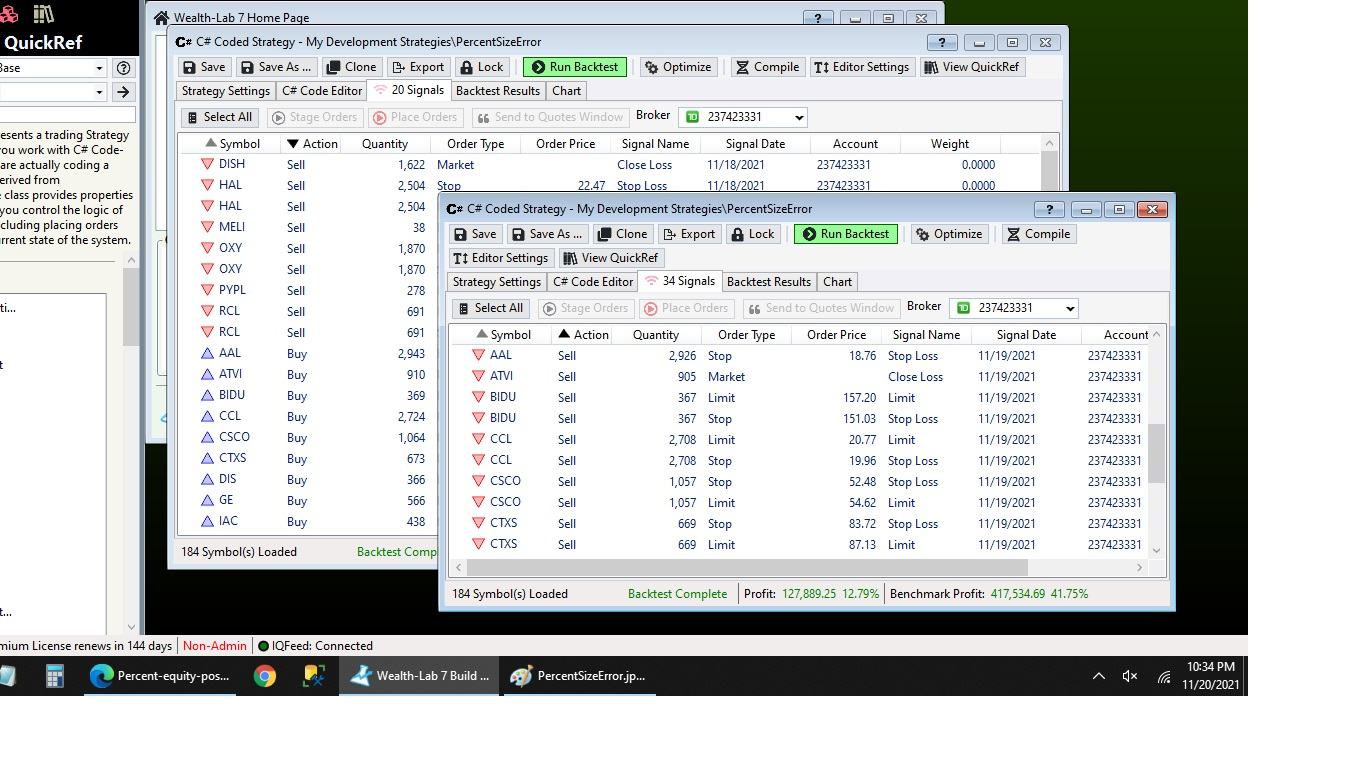And the settings used: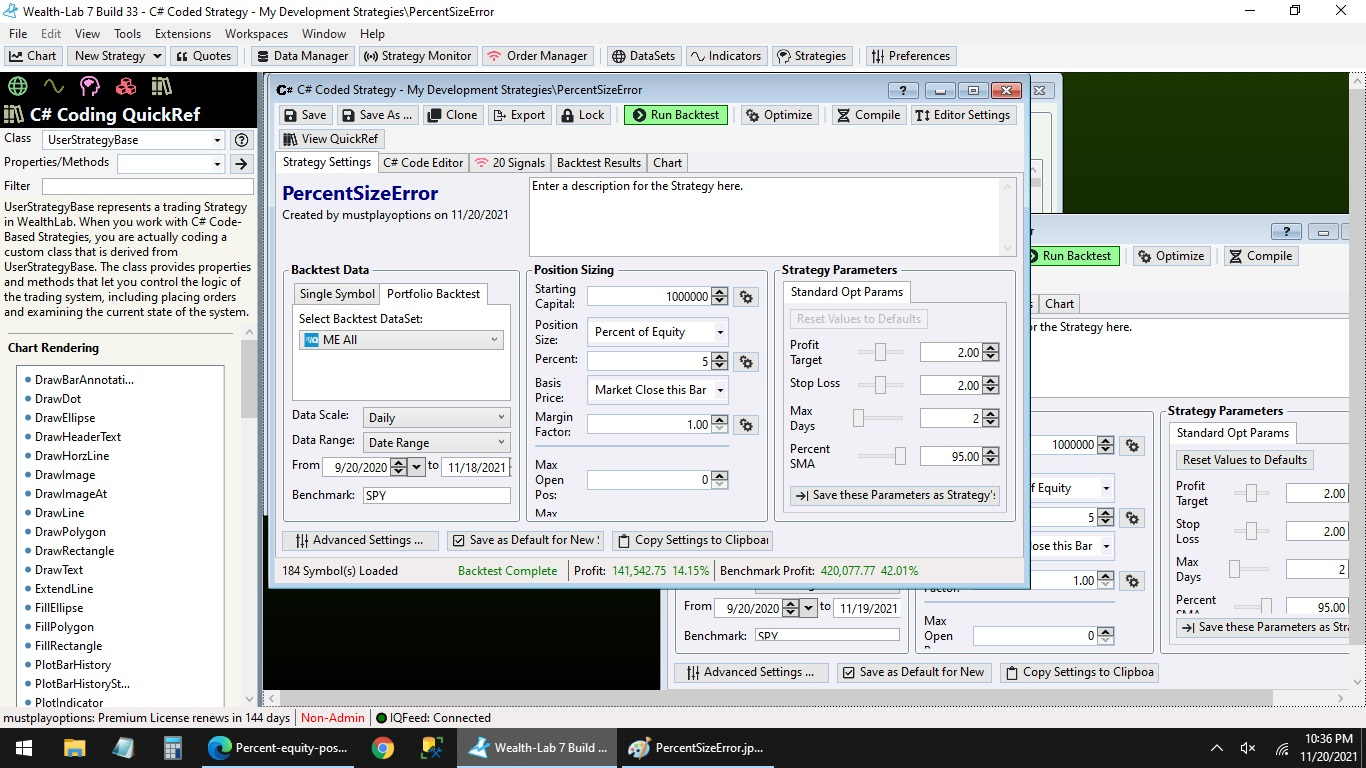My Portfolio Sync and Trading Threshold options are all unchecked in the Trading Preferences.

My broker is TD Ameritrade and my quote provider is IQFeed although in this situation those shouldn't effect anything.
0
Could it be that new positions are opened on Friday changing the overall picture?

P.S. A minor fix to the StartIndex:
```CODE://ignore data before the StartIndex
StartIndex = 14;
```
0
Almost certainly it's the [unimportant] result of the Trading Preference to "Use Brokerage-reported Account Value for % of Equity Position Size" for the Signals. Assume that Preference is selected...

Day 1:
Signals are generated based on the brokerage account value (say \$100K), not the backtest equity (say \$256K). If the % of equity size was 10%, you get a \$10K Position.

Day 2
You enter the \$10K position, which is what you want and re-run the backtest. Now you get an exit signal for about a \$25.6K Position. This is no problem if you also select either (or both) of the other Portfolio Sync Preferences to "Reduce Size of Exit Orders to match Position Quantity" and/or "Always Set Exit Order Quantity to full Position Quantity".

I guess you have not?
1
Image for reference -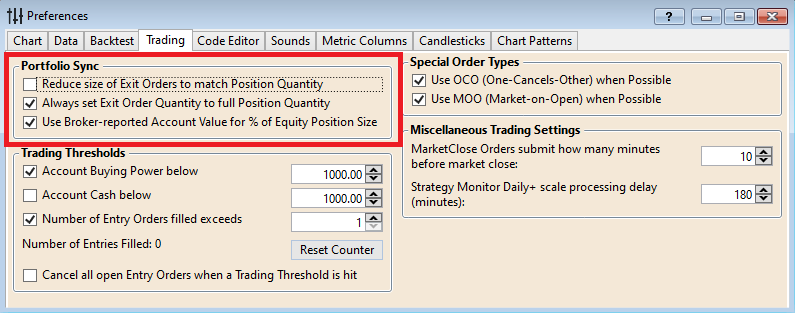0
Sorry that's not it. As I stated above those are all Unchecked.

BTW this is my core sample code not an actual strategy I run. I use it as a base for any new strategies since it has all my functions, kind of like a library, and replace the actual buy indicator/buy logic.
0
Eugene, that wouldn't surprise me at all but that would make it a bug as I suspect. How can values from Friday effect the signals from Thursday? They shouldn't.

And the other possibility I can think of that I mentioned is that the equity changes after each trade rather than using an equity from the beginning of the day when looking at position sizes from the day before.

The latter made me run the same strategy on 2 windows for the sales and both ending on 11/19/21 gave 2 different sell size signals. I'm not sure how this can't be a bug somewhere.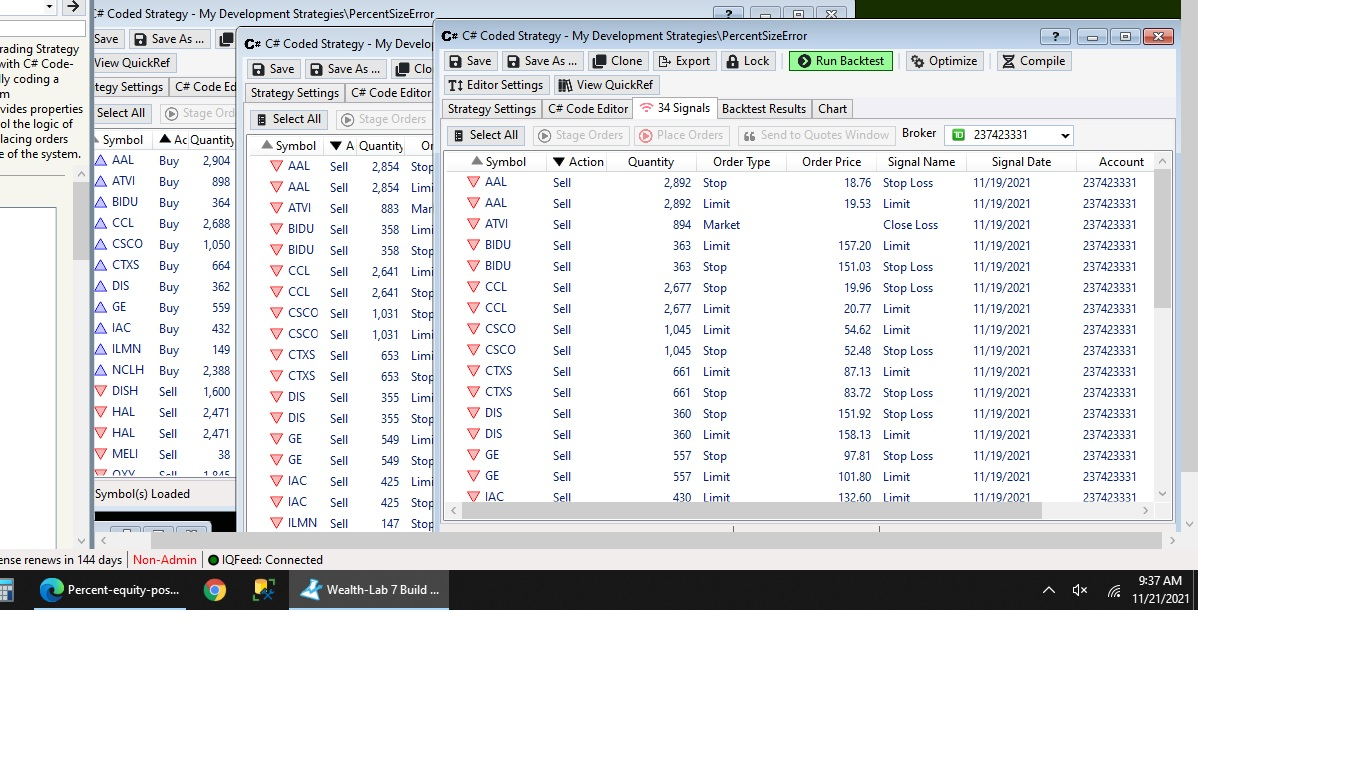In the pic the window on the left is the buy on 11/18/21. On the right BOTH windows end on 11/19/21. All 3 have different position sizes.
0
You can cry "bug" all you want, and I'd be the first person to jump to fix it, but you're not giving us anything to go on. The strategy code you provided doesn't generate any signals when I run it.

We need a single concrete, REPRODUCABLE case of symbol XYZ, for Strategy ABC, on X date showing incorrect quantity for its positon the following day.

I just ran ANOTHER run here and I'm not seeing the issue you describe at all. I WAS seeing some inconsistency, until I reduced my position size such that there were ZERO NSF positions in the metrics report. Double check your NSF positions. As far as I can see ... NO BUG.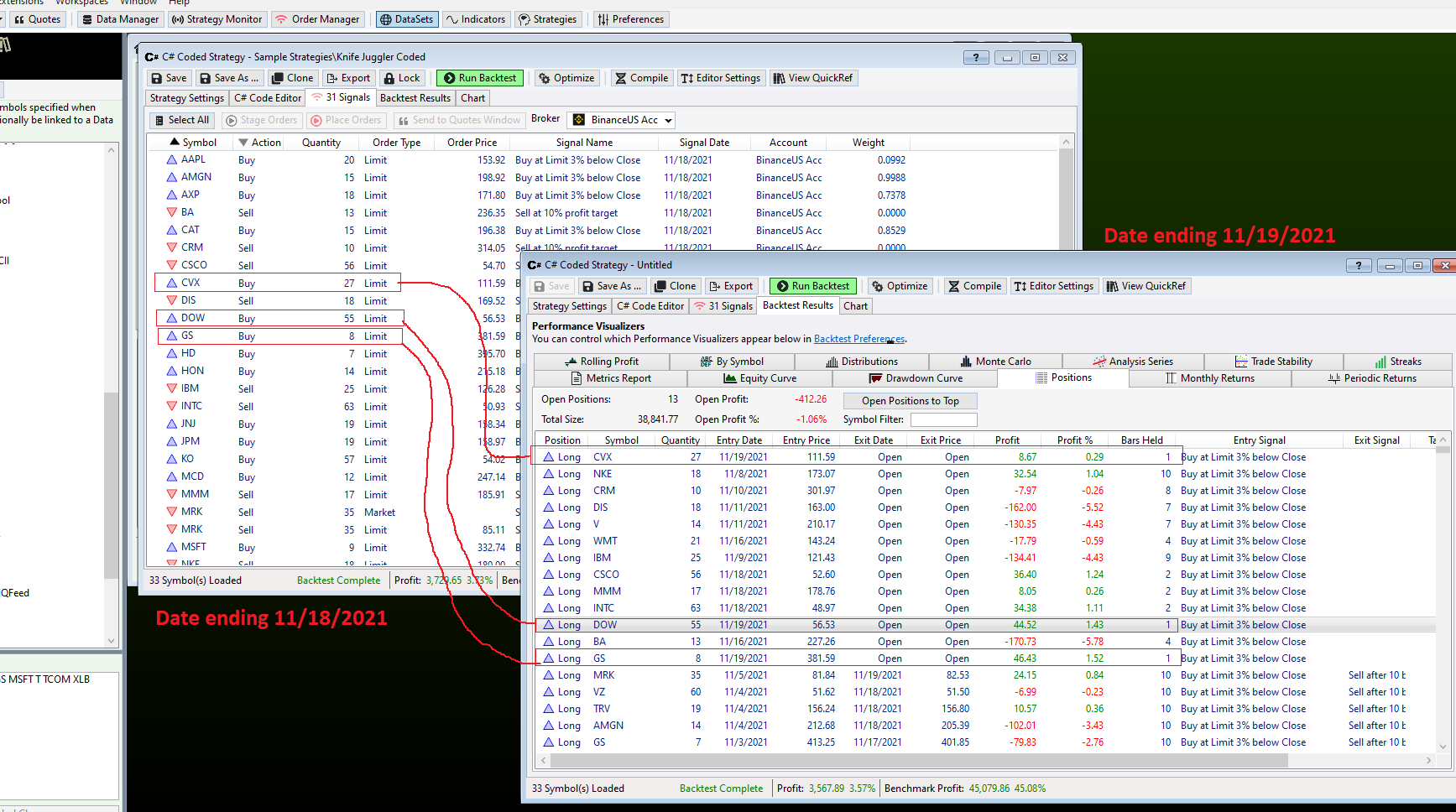0
Glitch,

What we have here seems to be a failure to communicate :)

Did you set the percent SMA to 95%? If that's too low it won't find signals. I just noticed in the code it didn't save that as the default.

The end result is what matters, not whether it's a bug or not. And I appreciate how responsive you have all been with issues that arise.

However, I am not sure what information is missing. I gave you sample code with sample dates and sample settings. I shared the prefs in the preferences. I showed pictures of the positions that give the "REPRODUCABLE case of symbol XYZ, for Strategy ABC, on X date showing incorrect quantity for its positon the following day." This happens every time I run the posted strategy with the settings described and pictured above.

The only thing I can think of left to do short of a screen share is the symbol list so here's the list I run the strategy on:

QUOTE:
A AA AAL AAPL ABBV ABT ACN ADBE ADI ADP ADSK AES AIG AKAM ALL ALXN AMAT AMGN AMZN ATVI AVGO AXP BA BAC BAX BIDU BIIB BK BKNG BLK BMY BSX C CAT CCL CERN CHKP CHTR CL CMCSA COF COP COST CSCO CSX CTAS CTSH CTXS CVS CVX DE DHR DIS DISCA DISH DLTR DUK EA EBAY EMR EXC EXPD EXPE F FB FDX FITB FLIR FLR GD GE GILD GM GOOGL GPS GS HAL HAS HD HIG HOLX HON HPQ HSIC IAC IBM IDXX ILMN INCY INTC INTU IP ISRG JBHT JD JNJ JPM KHC KLAC KMI KO LLY LMT LOW LRCX MA MAR MCD MCHP MDLZ MDP MDT MELI MET MMM MO MOS MRK MRO MS MSFT MSI MU MXIM NCLH NEE NFLX NKE NOC NTAP NTES NVDA ORCL ORLY OXY PAYX PCAR PEP PFE PG PM PYPL QCOM RCL REGN RHI ROST SBUX SIRI SLB SO SPG STT STX STZ SWKS T TEL TER TGT TMUS TROW TRV TSCO TSLA TXN UDR ULTA UNH UNP UPS USB V VRSK VRTX VZ WBA WDC WFC WMT WYNN XLNX XOM XRAY

The position size is 5%. As you can see in the picture there are only 11 open positions which is 55%. I don't see any NSF warnings - maybe I'm completely missing them? Usually I see a little warning next to the position or a message at the bottom.

Thanks for your persistence and patience with this.
0
Here. Let's try these settings. The other settings had NSF but not for that date. This way there are 0 NSF at all and there are still discrepencies.

The settings: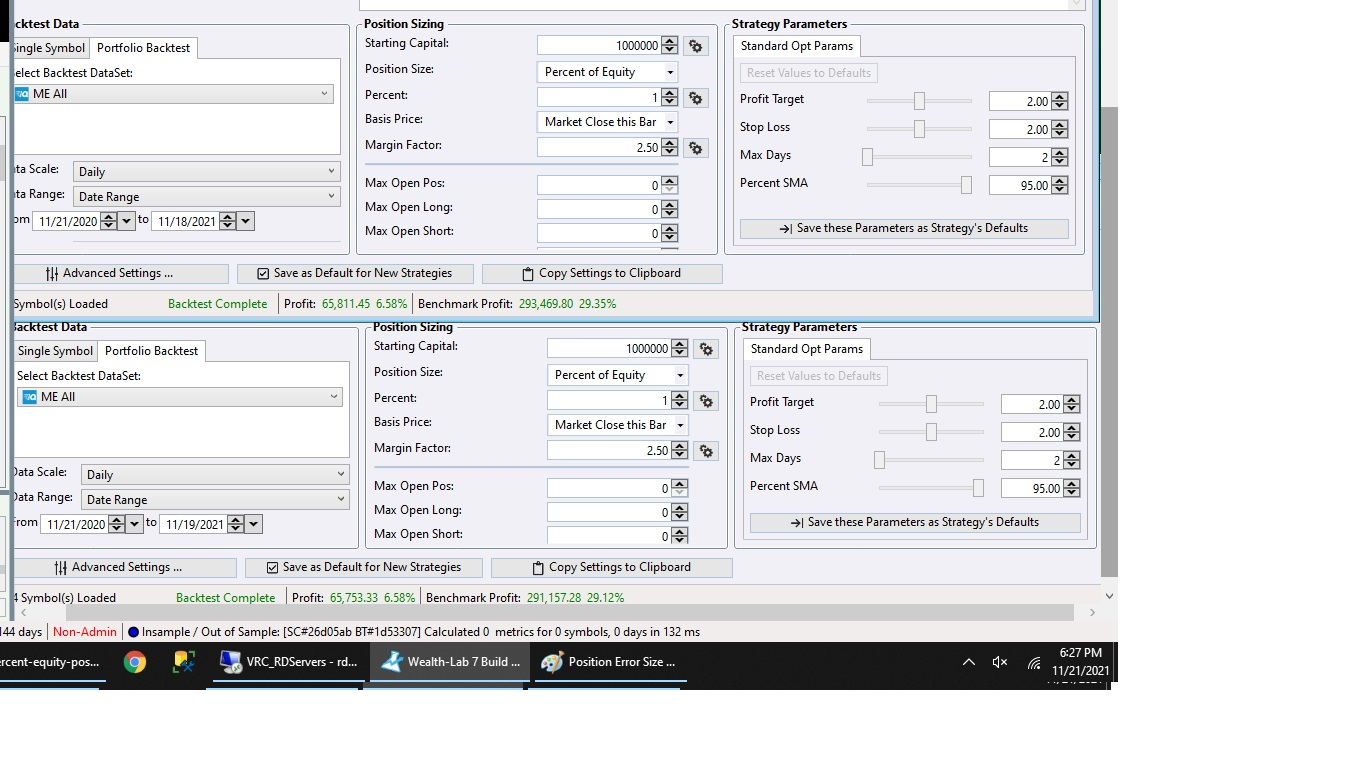No NSF: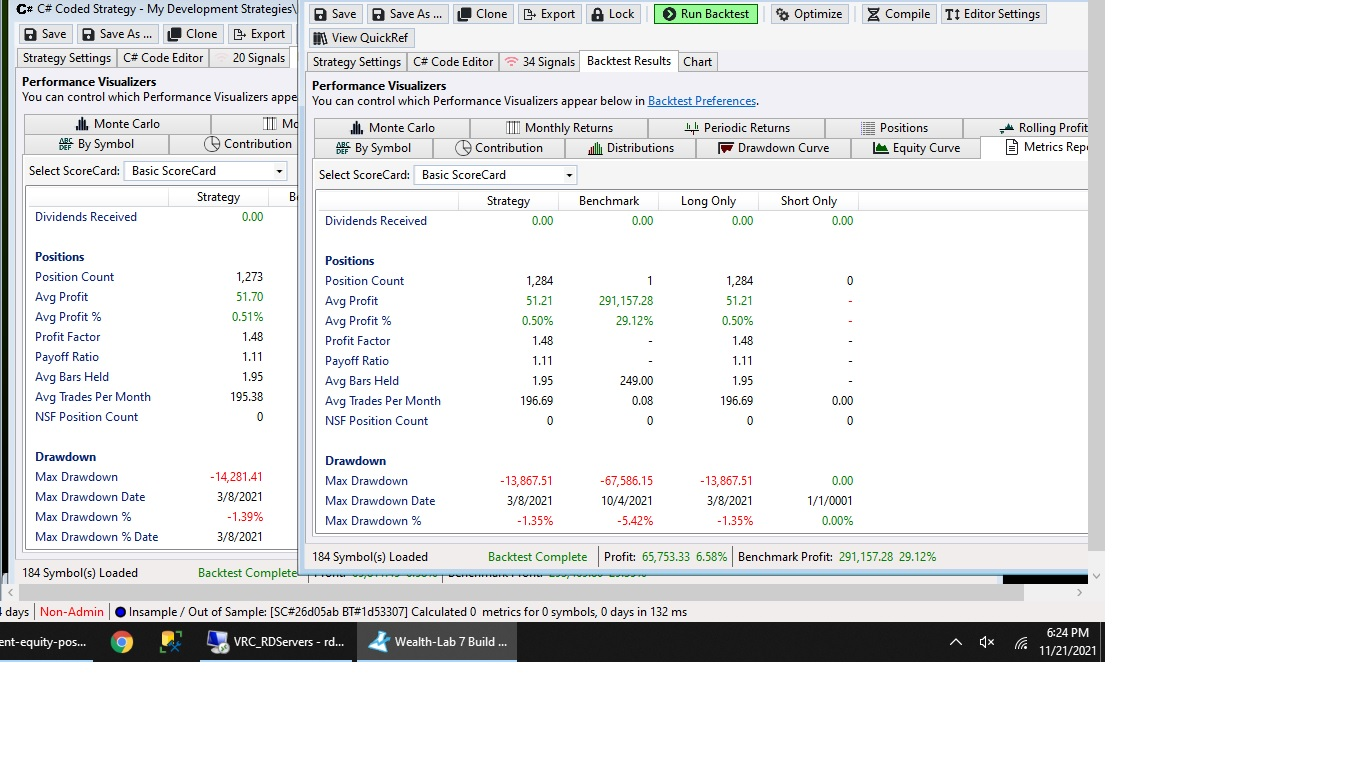Size Discrepency (smaller but still existent - Stocks AAL, CCL, NCLH):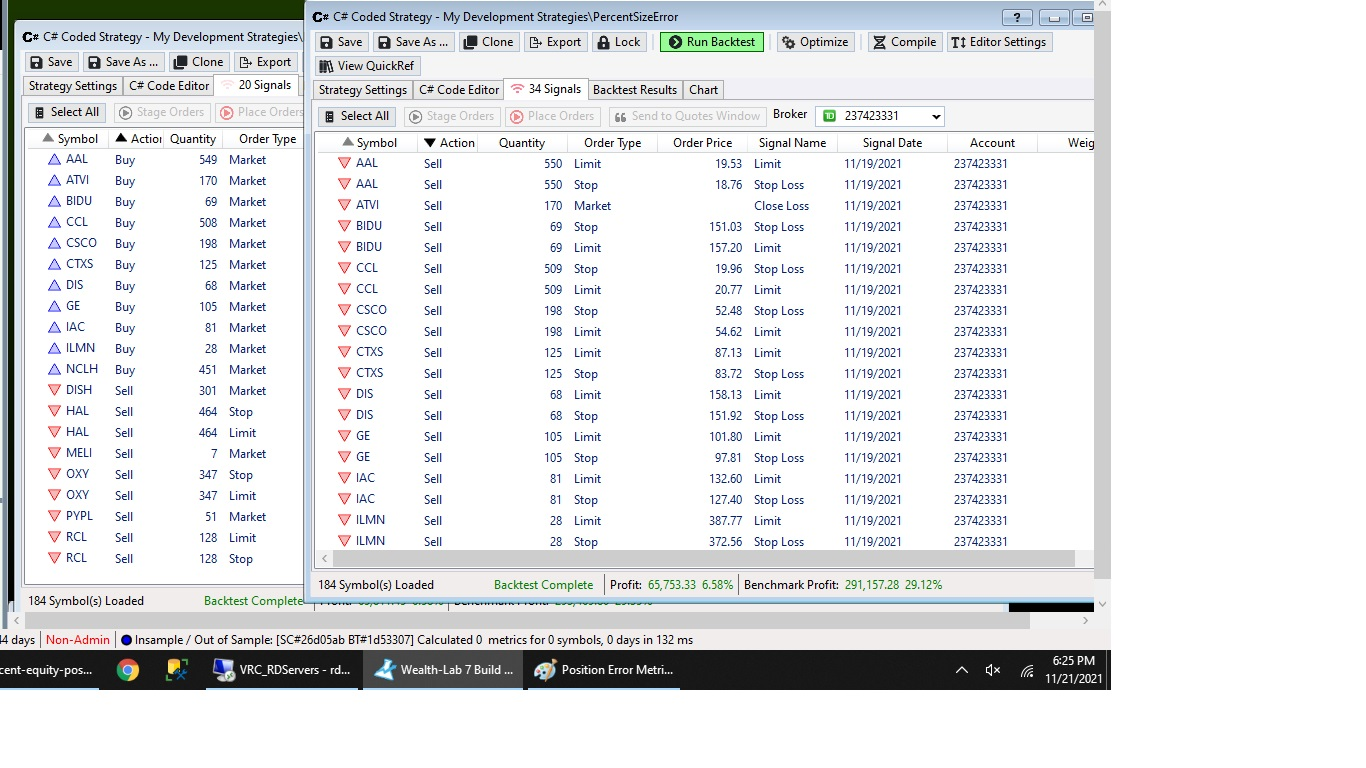I hope this helps.
0
I’ll try your code again tomorrow and change the parameter value. The NSFs I was speaking of would have been historical ones, reported in the metrics report. If they were present it would have potentially changed the backtest each run, accounting for any differences you might see.

Since you got the NSF count down to zero, you can see the discrepancy is one share at most, and this is likely a rounding difference but I’ll check it out more thoroughly tomorrow.
0
Even if it's a rounding error though then we still have to manually change orders to match the position sizes. Hopefully that's all it is and it's an easy fix.

Thank you!
0
Well it took about an hour of painstaking analysis, but I discovered why your runs are not consistent. Your Strategy issues two sell orders, one stop and one limit. There are some cases where both of them can hit in a single bar, and in these cases WL7 determines which executes randomly. This leads to slightly different equity curves each time and so inconsistent signal quantity.

Now, because of subtle real world issues like this it's why I recommend not using percent of equity position sizing for a Strategy you're actually trading. You know your desired position size each day, just set it. Or, just use the Portfolio Sync feature to automatically set the quantity to whatever the size of your actual position is.

Good to know it wasn't a bug!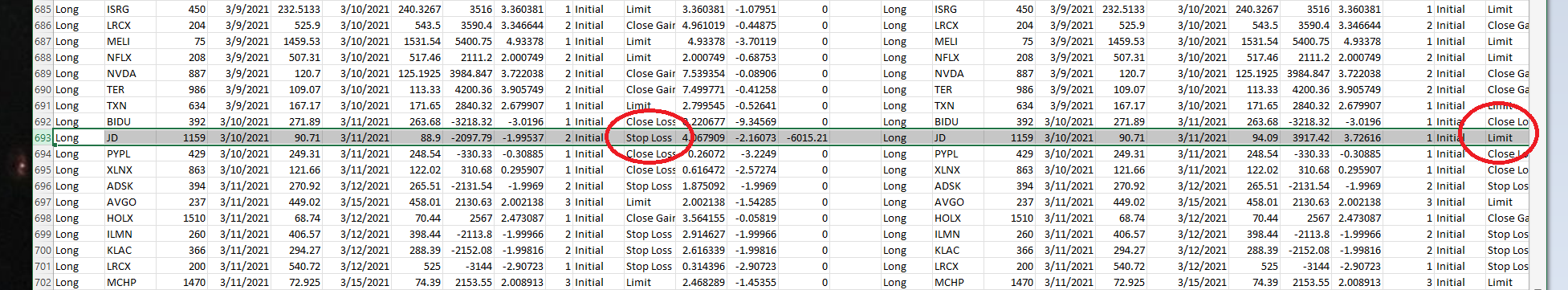1
I'm glad it's not a bug too. Sorry for the time but I really appreciate the explanation.

So on 11/18 one of the open positions could've taken a gain or loss and that's why?

I'll try those settings and see what happens.

Thank you very much

0
No worries, it's important to understand what's going on when difficult to explain things like this happen. It also exposed a small gap we have in the framework. ClosePosition could easily return the Transaction instance, just like PlaceTrade. You could then assign a Weight, which would eliminate the effect. Additionally, we could introduce a setting to handle cases like this in a consistent manner.

But for now as a workaround you can use this slightly modified version which grabs the resulting Transaction and assigns a Weight, favoring the stop loss over the limit exit.

```CODE:
using WealthLab.Backtest;
using System;
using WealthLab.Core;
using WealthLab.Indicators;
using System.Drawing;
using System.Collections.Generic;

namespace WealthScript2
{
public class HelloWorldStrategy : UserStrategyBase
{
//declare parameter variables
int max_days;
double profit_target, stop_loss;
int percent_sma;

//declare other variables

//time series variables are used for calculated bar data
TimeSeries sma_values;

//the base procedure must have the same name as the class
public HelloWorldStrategy() : base()
{
AddParameter("Profit Target", ParameterTypes.Double, 2, 1, 3, 1);
AddParameter("Stop Loss", ParameterTypes.Double, 2.0, 1, 3, 0.5);
AddParameter("Max Days", ParameterTypes.Int32, 2, 2, 5, 1);
AddParameter("Percent SMA", ParameterTypes.Int32, 95, 5, 100, 5);

}

//create indicators and other objects here, this is executed prior to the main trading loop
//also do plotting here
public override void Initialize(BarHistory bars)
{

//assign parameters to variables
profit_target = (double)Parameters.Value;
stop_loss = (double)Parameters.Value;
max_days = (int)Parameters.Value;
percent_sma = (int)Parameters.Value;

//create new series that can be pre-calculated here
sma_values = SMA.Series(bars.Close, 14);

//create new series that need to be calculated here

//ignore data before the StartIndex
StartIndex = 1;

//put headers on the chart here

//do all the plotting of anything that can be pre-calculated here
//   plot anything that needs to be calculated below in Cleanup
PlotTimeSeries(sma_values, sma_values.Description, "Price", Color.Red, PlotStyles.Line);

}

//color the background based on the level of profit/loss
public void WinLossBackground(BarHistory bars, int idx)
{
int profit_level = 0;
System.Drawing.Color color;
// use code_s to find the worst trade for overlapping positions
double profit = 0.0, code_s = 0.0;

// First determine the worst profit level at each bar based on the positions
List<Position> positions = GetPositions();

foreach (Position p in positions)
{
// foreach (Position p in OpenPositions) won't work because it skips the ExitBar
if (!(p.IsOpen || idx == p.ExitBar))
continue;

//can't use p.Profit because the profit isn't calculated yet so it just gives open cost
profit = p.ProfitPctAsOf(idx);

// Profit/Loss in percent
if ((profit > 5))
profit_level = (int)-1;
else if ((profit > 0.5))
profit_level = (int)-2;
else if ((profit > -0.5))
profit_level = (int)-3;
else if ((profit > -5))
profit_level = (int)-4;
else
// profit <= -5.0
profit_level = (int)-5;

double old_code = 0.0;

// keep most pessimistic in overlapping trades
old_code = (double)code_s;
if ((profit_level < old_code))
code_s = profit_level;
} // foreach position

// Now set the background color for the bar
int color_code = 0;

color_code = (int)Math.Floor(code_s);
switch (color_code)
{
case -1:
color = Color.LimeGreen;
break;
case -2:
color = Color.LightGreen;
break;
case -3:
color = Color.LightYellow;
break;
case -4:
color = Color.MistyRose;
break;
case -5:
color = Color.LightPink;
break;
default:
color = Color.White;
break;
} // switch   color_code

SetBackgroundColor(bars, idx, color);
} // WinLossBackground

double RoundPrice(double price, double decimal_points)
{
return Math.Round(price * Math.Pow(10, decimal_points)) / Math.Pow(10, decimal_points);
}

//use to fix price to sell short or buy long
double FloorPrice(double price, double decimal_points)
{
return Math.Floor(price * Math.Pow(10, decimal_points)) / Math.Pow(10, decimal_points);
}

//use to fix price to sell long or buy short
double CeilingPrice(double price, double decimal_points)
{
return Math.Ceiling(price * Math.Pow(10, decimal_points)) / Math.Pow(10, decimal_points);
}

public void SellStrategy(BarHistory bars, int idx, double ProfitTarget, int days)
{
//go through each position and sell
foreach (Position p in OpenPositions)
{
// Set Close signals
if (p.PositionType == PositionType.Long)
{
//End trade after a fixed number of days
if ((idx + 1) - p.EntryBar > days)
ClosePosition(p, OrderType.Stop, bars.Close[idx] - 0.01, "DaysExpired");
else
{
if (bars.Close[idx] > (double)(p.EntryPrice * (1 + profit_target / 100.0)))
ClosePosition(p, OrderType.Stop, bars.Close[idx] - 0.01, "Close Gain");
else
{
if (bars.Close[idx] < (double)(p.EntryPrice * (1 - stop_loss / 100.0)))
ClosePosition(p, OrderType.Market, 0, "Close Loss");
else
{
//when placing both orders this can be a conditional one-cancels-other
ClosePosition(p, OrderType.Limit, CeilingPrice(p.EntryPrice * (1 + profit_target / 100.0), 2), "Limit");
Transaction t = Backtester.TransactionLog[Backtester.TransactionLog.Count - 1];
t.Weight = 0.5;
ClosePosition(p, OrderType.Stop, CeilingPrice(p.EntryPrice * (1 - stop_loss / 100.0), 2), "Stop Loss");
t = Backtester.TransactionLog[Backtester.TransactionLog.Count - 1];
t.Weight = 1.0;
}
}
} // if expired long
} // if long
else
{
if ((idx + 1) - p.EntryBar > days)
ClosePosition(p, OrderType.Stop, bars.Close[idx] + 0.01, "DaysExpired");
else
{
if (bars.Close[idx] > (double)(p.EntryPrice * (1 - profit_target / 100.0)))
ClosePosition(p, OrderType.Stop, bars.Close[idx] + 0.01, "Close Gain");
else
{
if (bars.Close[idx] < (double)(p.EntryPrice * (1 + stop_loss / 100.0)))
ClosePosition(p, OrderType.Market, 0, "Close Loss");
else
{
//when placing both orders this can be a conditional one-cancels-other
ClosePosition(p, OrderType.Limit, FloorPrice(p.EntryPrice * (1 - profit_target / 100.0), 2), "Limit");
Transaction t = Backtester.TransactionLog[Backtester.TransactionLog.Count - 1];
t.Weight = 0.5;
ClosePosition(p, OrderType.Stop, FloorPrice(p.EntryPrice * (1 + stop_loss / 100.0), 2), "Stop Loss");
t = Backtester.TransactionLog[Backtester.TransactionLog.Count - 1];
t.Weight = 1.0;
}
}
} // if expired short
} // if short
} // OpenPositions
} // WaveRiderSell

public void BuyStrategy(BarHistory bars, int idx)
{
if ((OpenPositions.Count == 0) && (bars.Close[idx] < (percent_sma / 100.0) * sma_values[idx]))
}

public override void Cleanup(BarHistory bars)
{
//do all the plotting of any series that need to be calculated by bar here
}

//execute the strategy rules here, this is executed once for each bar in the backtest history
public override void Execute(BarHistory bars, int idx)
{

// put the sell orders or sell procedure here
SellStrategy(bars, idx, profit_target, max_days);

//calculate series that need to be calculated by bar in Execute

//change background colors by bar here
WinLossBackground(bars, idx);

} // Execute

} // class
} // namespace
```
2
Ha! I was going to ask for a preference to take the worse of the 2 and you already suggested it AND gave a workaround. That's perfect thank you.

I'll implement this and hope my results aren't actually so bad as to negate the strategy that I thought was profitable.

Thanks!
0
The event is so rare that it shouldn’t materially impact your results, let me know if it causes the differences to cease.
0
Evidently it's not that rare at all.

It took a 14M in 10 year strategy down to 1.5M and changed 2 profitable years to significant losses. No wonder my backtesting hadn't been matching my real life trades nearly as closely as I thought they should!

Thanks for figuring it out. Now I have to figure out if it's worth continuing the strategy or not.
0